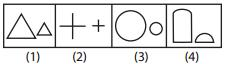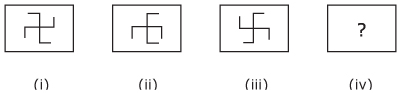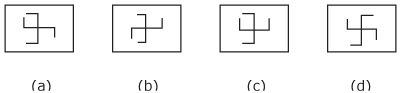# UPSC 2019: Practice Questions for IAS Prelims GS II CSAT Day 6

The UPSC civil services prelims exam is due to be held on 2nd June 2019. If you are a serious IAS aspirant, you would be busy preparing for both the GS paper I and II. Solving sample papers is a very important part of your UPSC preparation as it helps you in assessing your strengths and weaknesses. So, we bring you a series of sample questions for your practice. This article gives you ten model CSAT questions.

Try the following sample CSAT questions 2019:

Q1. A person travels 12 km due North, then 15 km due East, after that 15 km due west and then 18 km due south. How far is he from the starting point?

1. 12 km
2. 6 km
3. 15 km
4. 10 km

Q2. A person has 4 coins each of different denominations. What is the number of different sums of money the person can form (using one or more coins at the time)?

1. 15
2. 12
3. 16
4. 10

Q3. There are four persons A, B, C, D and A has some coins. A gave half of the coins to B and 4 more besides. B gave half of the coins to C and 4 more besides. C gave half of the coins to D and 4 more besides. Both B and D end up with same number of coins. How many coins did A have originally?

1. 98
2. 50
3. 72
4. 64

Q4. A train 360 m long is running at a speed of 45 km/hr. In what time will it pass a bridge 140 m long?

1. 45 s
2. 40 s
3. 36 s
4. 50 s

Q5. In the following question, a number series is given with one term missing. Choose the correct alternative that will continue the same pattern and fill in the blank spaces.

1, 9, 25, 49, 81, ?

1. 100
2. 144
3. 125
4. 121

Q6. Twenty women can do a work in sixteen days. Sixteen men can complete the same work in fifteen days. What is the ratio between the capacity of a man and woman?

1. 4:3
2. 5:3
3. 6:7
4. 2:3

Q7. How many numbers lie between 300 and 500 in which 4 comes only one time?

1. 100
2. 110
3. 90
4. 99

Q8. Choose the figure which is different:1. 1
2. 2
3. 3
4. 4

Q9. There are three cans A, B and C. The capacities of A, B and C are 6 litres, 10 litres and 16 litres respectively. The can C contains 16 litres of milk. The milk has to be divided in them using these three cans only.

Consider the following statements:

1. It is possible to have 6 litres of milk each in can A and can B.
2. It is possible to have 8 litres of milk each in can B and can C.

Which of the statements given above is/are correct?

1. 1 only
2. 2 only
3. Both 1 and 2
4. Neither 1 nor 2

Q10. Which figure will replace the question mark?a) a
b) b
c) c
d) d

Check the answer key for the above questions:

```1. B   2. A    3. C    4. B    5. D    6. A     7. D    8. D     9. C    10. C
Also read-
How to read the Hindu?
```